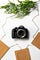# Object-Oriented JavaScript — StringsPhoto by Rajiv Perera on Unsplash

JavaScript is partly an object-oriented language.

To learn JavaScript, we got to learn the object-oriented parts of JavaScript.

In this article, we’ll look at strings, which is one of the building blocks of objects.

# Strings

A string is a sequence of characters to represent text.

Any values placed between single quotes, double quotes, or backticks are string.

If we have:

`let s = "foo";typeof s;`

Then `typeof s` returns `'string'` .

If we put nothing between the quotes, it’s still a string.

The `+` operator is used to concatenate 2 strings.

If we have:

`let s1 = "web";let s2 = "site";let s = s1 + s2;`

Then we `s` is `'website'` .

And `typeof s` would be `'string'` .

This is a source of errors in the system.

To avoid errors, we should make sure that operators are strings.

This way, we won’t be adding anything by accident.

# String Conversions

A string is converted to a number behind the scene if a number string is encountered.

For instance, if we have:

`let s = '1';s = 2* s;`

Then `typeof s` returns `'number'` .

If we have:

`let s = '1';s++;`

Then `s++` converts `s` to `'number'` .

We can convert a number string to a number by multiplying y 1 or use `parseInt` .

For instance, we can write:

`let s = "100";s = s * 1;`

Now `s` is a string.

If the conversion fails, we get `NaN` .

There’re many strings with special meanings.

`\` is the escape character.

We can use it to escape `\`, `'` and `"` so that they can be in th string.

For instance, we can write:

`const s = "1\\2";`

and `s` is `1 \ 2` .

`\n` is the line end character.

If we have:

`let s = ‘\n1\n2\n3\n’;`

We get:

`"123"`

`\r` is the carriage return character.

If we have:

`let s = '1\r2'`

We get:

`“12”`

`\t` is the tab character. If we have:

`let s = "1\t2";`

We get:

`"1 2"`

`\u` is the character code that lets us use Unicode.

For instance, we can write:

`let s = 'e\u0301'`

and `s` is

`'é'`

# String Template Literals

ES6 introduced the template literal.

They let us embed expressions within regular strings.

ES6 has template literals and tagged literals.

Template literals are single or multiline strings with embedded expressions.

For instance, we can write:

`const level = "debug";const message = "meltdown";console.log(`level: \${level} - message: \${message}`)`

We have the `level` and `message` variables embedded into the template literals.

So we get:

`'level: debug - message: meltdown'`

logged.

Template literals are surrounded by backtick characters instead of quotes.

They’re concatenated into a single string.

We can have any expression int eh `\${}` .

For instance, we can write:

`const a = 10;const b = 10;function sum(x, y) {  return x + y}function multiply(x, y) {  return x * y}console.log(`sum is \${sum(a, b)} and product is \${multiply(a, b)}.`);`

We embed the result of the function calls into the template literal.

And we get:

`'sum is 20 and product is 100.'`

returned.Photo by Amrit Sangar on Unsplash

# Conclusion

Strings are a sequence of characters.

We can create strings and template literals, which can have expressions embedded in them.

Web developer. Subscribe to my email list now at https://thewebdev.info/subscribe/. Email me at hohanga@gmail.com

Web developer. Subscribe to my email list now at https://thewebdev.info/subscribe/. Email me at hohanga@gmail.com